• 转置卷积又被称为反卷积和逆卷积，但是转置卷积才是最正规和主流的叫法，在主流的深度学习框架中，转置卷积的函数名都是conv_transpose 因为转置卷积的运算过程很容易让人误解，举一个例子，一个4*4的输入经过3*3的...
前言
转置卷积又被称为反卷积和逆卷积，但是转置卷积才是最正规和主流的叫法，在主流的深度学习框架中，转置卷积的函数名都是conv_transpose
因为转置卷积的运算过程很容易让人误解，举一个例子，一个4*4的输入经过3*3的卷积核(stride=1,padding=0)输出为2*2，而转置卷积将这个输出当作输入，即一个2*2的输入经过3*3的转置卷积核输出为4*4，看起来很像是卷积的逆操作，但事实并不是一个逆过程的概念
转置卷积
用公式可以更好的说明转置卷积与逆卷积的不同
卷积运算可以这么表示：
$y = Cx$
如果按照线性代数中的逆矩阵的概念，逆卷积的公式应该是这样：
$x = C^{-1}y$
但是转置卷积的真实公式是：
$x = C^{T}y$
参考https://zhuanlan.zhihu.com/p/79680474给出的用Numpy实现转置卷积，可以更直观的理解
from torch import nn
import numpy as np
import torch
batch_size = 1
stride = 1
padding = "VALID"
input_channel = 1
input_size = 5
output_channel = 1
filter_size = 3
output_size = 3

input_np = np.reshape(np.arange(input_size*input_size, dtype="float32"),newshape=[input_size,input_size])
print(f"input_np = {input_np}")
print("input_up.shape = %s" % str(input_np.shape))

input_np_flattern = np.reshape(input_np, newshape=[input_size*input_size, 1])
print(f"input_np_flattern = {input_np_flattern}")
print("input_np_flattern = %s" % str(input_np_flattern.shape))

filter_np = np.reshape(np.arange(filter_size*filter_size, dtype="float32"),newshape=[filter_size,filter_size])
print(f"filter_np = {filter_np}")
print("filter_np.shape = %s" % str(filter_np.shape))

filter_np_matrix = np.zeros((output_size,output_size,input_size,input_size))
print(filter_np_matrix.shape)
# 卷积
for h in range(output_size):
for w in range(output_size):
start_h = h*stride
start_w = w*stride
end_h = start_h + filter_size
end_w = start_w + filter_size
filter_np_matrix[h, w, start_h:end_h, start_w:end_w] = filter_np
filter_np_matrix = np.reshape(filter_np_matrix,newshape=[output_size*output_size, input_size*input_size])
print(f"filter_np_matrix = {filter_np_matrix}")
print("filter_np_matrix.shape = %s" % str(filter_np_matrix.shape))
# 相乘
output_np = np.dot(filter_np_matrix, input_np_flattern)
output_np = np.reshape(output_np, newshape=[output_size, output_size])
print(f"output_np = {output_np}")
print("output_np.shape = %s" % str(output_np.shape))

# 反卷积
output_np_flattern = np.reshape(output_np, newshape=[output_size*output_size, 1])
output_np_transpose = np.dot(filter_np_matrix.T, output_np_flattern)
output_np_transpose = np.reshape(output_np_transpose, newshape=[input_size,input_size])
print(f"output_np_transpose = {output_np_transpose}")
print("output_np_transpose.shape = %s" % str(output_np_transpose.shape))

用PyTorch实现转置卷积
仿照沐神的动手深度学习一书，用PyTorch重写了下
# 初始化权重
conv_weight = torch.arange(1,17,dtype=torch.float32).reshape((1,1,4,4))
conv_input = torch.arange(1,10,dtype=torch.float32).reshape((1,1,3,3))
conv = nn.Conv2d(in_channels=1, out_channels=1, kernel_size=3, padding=0)
print(conv.weight)

conv.weight = torch.nn.Parameter(conv_weight)
print(conv.weight)
print(conv.forward(conv_input))
# 用矩阵乘法实现卷积运算
W, k = torch.zeros((4,16)) , torch.zeros(11)
k[:3], k[4:7], k[8:] = conv_input[0, 0, 0, :], conv_input[0, 0, 1, :], conv_input[0, 0, 2, :]
W[0, 0:11], W[1, 1:12], W[2, 4:15], W[3, 5:16] = k, k, k, k
print(torch.mm(W,conv_input.reshape(16,-1)).reshape((1,1,2,2)))

# conv2d
conv = nn.Conv2d(in_channels=3, out_channels=10, kernel_size=4, padding=1, stride=2)
conv_weight = torch.arange(0,480,dtype=torch.float32).reshape((10,3,4,4))
conv.weight = torch.nn.Parameter(conv_weight)   # 初始化conv

print(f"conv_weight_shape={conv_weight.shape}")
print(conv)
conv_input = torch.randn((1,3,64,64),dtype=torch.float32)
conv_output = conv.forward(conv_input)
print(f"conv_output={conv_output.shape}")  # 经过conv2d之后，原图尺寸缩小了两倍
# transpose conv
conv_trans = nn.ConvTranspose2d(in_channels=10,out_channels=3,kernel_size=4,padding=1,stride=2)
conv_trans_weight = torch.arange(0,480,dtype=torch.float32).reshape((3,10,4,4))
conv_trans_weight.weight = torch.nn.Parameter(conv_trans_weight)   # 初始化conv
print(f"conv_trans_weight_shape={conv_trans_weight.shape}")
print(f"conv_trans_output={conv_trans.forward(conv_output).shape}")




展开全文深度学习 numpy 神经网络
• 大家好，新手入门语义分割，在利用pytorch实现Linknet的时候，在编码层最后输出的尺寸为6×10（上一层为12× 20），文中规定反卷积核的尺寸为k为3× 3，步长s = 2，利用反卷积求输出尺寸的大小为N（out） = （N（in...python 深度学习
• 反卷积不同的是，反卷积是先补零，在进行卷积操作，而亚像素卷积是直接对数据进行channel，维度的扩大，再通过reshape的方式来提高分辨率。 亚像素卷积走的是： [B,H,W,C] -》[B,H,W,Crr] -》[B,Hr,Wr,C] 的过程，...
前言
作为一种增强数据分辨率的方法，亚像素卷积被用于计算机视觉中的超分任务当中。与反卷积不同的是，反卷积是先补零，在进行卷积操作，而亚像素卷积是直接对数据进行channel，维度的扩大，再通过reshape的方式来提高分辨率。
亚像素卷积走的是：
[B,H,W,C] -》[B,H,W,Crr] -》[B,Hr,Wr,C] 的过程，集体流程如下图所示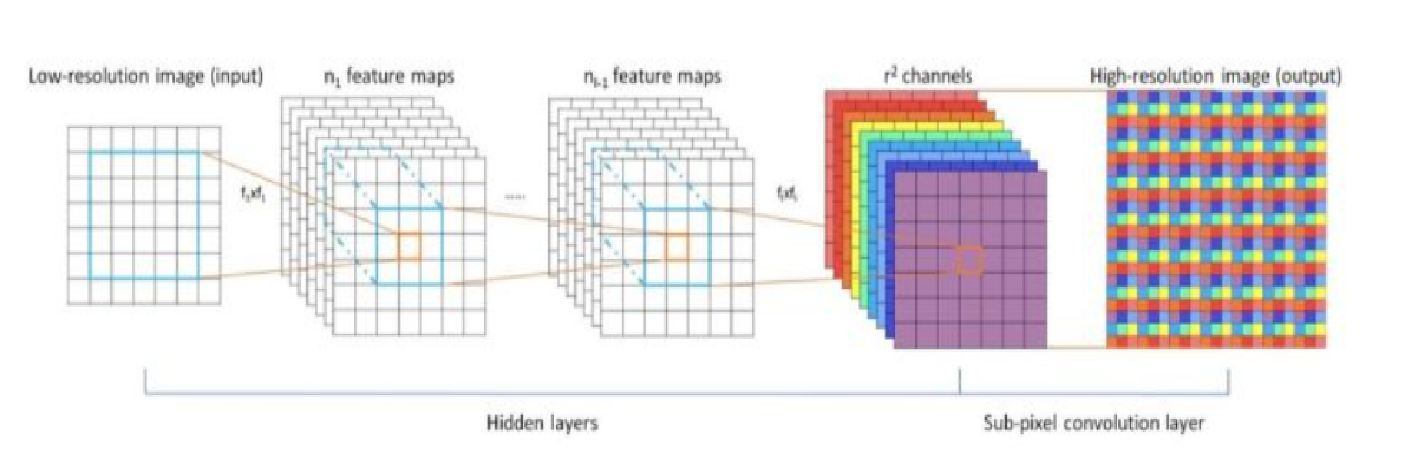代码实现
import torch
import torch.nn as nn
import torch.nn.functional as F

class oneDConv(nn.Module):
# 卷积+ReLU函数
def __init__(self, in_channels, out_channels, kernel_sizes, paddings, dilations):
super().__init__()
self.conv = nn.Sequential(
nn.Conv1d(in_channels, out_channels, kernel_size=kernel_sizes , padding = paddings, dilation = dilations),
nn.BatchNorm1d(out_channels),
#nn.LayerNorm([out_channels,3199]),
)

def forward(self, x):
x = self.conv(x)
return x

class SubConv(nn.Module):
# 卷积+ReLU函数
def __init__(self, in_channels, r, kernel_sizes, paddings, dilations):
super().__init__()
self.conv = nn.Sequential(
nn.Conv1d(in_channels, in_channels*r, kernel_size=kernel_sizes , padding = paddings, dilation = dilations),
nn.BatchNorm1d(in_channels*r),
#nn.LayerNorm([out_channels,3199]),
)

def forward(self, x):
B,C,T = x.size()
print(B,C,T)
x = self.conv(x)
x = torch.reshape(x,(B,C,-1)) ###变形
return x
x=torch.randn(5, 1, 32000)

net = SubConv(1,2,1,0,1)
outputs = net(x)
print(outputs.size())

结果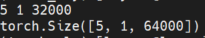展开全文• 这个参数在做步长为1的反卷积时是不用在意的。 然而当步长大于1了，就需要手动设置以免网络出错。 1.思考卷积过程 因为例如在valid模式下 7×7的输入尺寸+步长为2+卷积核3×3 = 3×3输出尺寸。 8×8的输入尺寸+步长...
nn.ConvTranspose2d()
在传入参数时除了常见的输入输出通道数、卷积核尺寸等，还会有一个稍微令人费解的参数：output_padding
这个参数在做步长为1的反卷积时是不用在意的。
然而当步长大于1了，就需要手动设置以免网络出错。
1.思考卷积过程
因为例如在valid模式下

7×7的输入尺寸+步长为2+卷积核3×3 = 3×3输出尺寸。
8×8的输入尺寸+步长为2+卷积核3×3 = 3×3输出尺寸。
因为使用了 地板除(floor)这个原则实现

2.反卷积过程
3×3 的输入进行步长为2的反卷积，7×7的输出与8×8的输出都将会是合法的。
output_padding参数作用

接下来我们再给出反卷积尺寸变化计算公式，根据公式计算参数值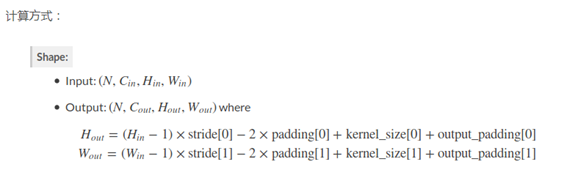由于卷积核滑动过程中，边界情况的不确定，使得在运算步长大于1的反卷积时会出现多种合法输出尺寸，pytorch的反卷积层提供了output_padding供使用者选择输出，一般情况下我们希望输入输出尺寸以步长为比例，因此output_padding一般取stride-1，同时padding取 (kernel_size - 1)/2 。
链接：https://blog.csdn.net/qq_41368247/article/details/86626446


展开全文• 转置卷积（transposed convolution）是一种上采样技术，操作过程是卷积的反过程，也被称作反卷积（deconvolution），但它的操作结果不是卷积的逆。它也可以通过卷积操作来实现，只是需要将卷积核旋转180度。它主要...
前言

原先是将这篇笔记和上一篇笔记合起来写的，但是由于内容很多，于是将卷积与转置卷积分作两篇。转置卷积（transposed convolution）是一种上采样技术，操作过程是卷积的反过程，也被称作反卷积（deconvolution），但它的操作结果不是卷积的逆。它也可以通过卷积操作来实现，只是需要将卷积核旋转180度。它主要应用在图像分割和超分辨率等任务中。笔记主要包括转置卷积操作和Pytorch转置卷积层。本笔记的知识框架主要来源于深度之眼，并依此作了内容的丰富拓展，拓展内容主要源自对torch文档的翻译，对孙玉林等著的PyTorch深度学习入门与实战的参考和自己的粗浅理解，所用数据来源于网络。发现有人在其他平台照搬笔者笔记，不仅不注明出处，有甚者更将其作为收费文章，因此笔者将在文中任意位置插入识别标志。
笔记是笔者根据自己理解一字一字打上去的，还要到处找合适的图片，有时为了便于理解还要修图，原创不易，转载请注明出处，文中笔者哪怕是引图也注明了出处的
结果可视化见：深度之眼Pytorch打卡（十）：Pytorch数据预处理——数据统一与数据增强（上）
卷积操作见：深度之眼Pytorch打卡（十四）：Pytorch卷积神经网络部件——卷积操作与卷积层、转置卷积操作与转置卷积层（反卷积）（对卷积转置卷积细致动图分析）

转置卷积操作（transposed convolution）

转置卷积

转置卷积有时被称作反卷积，结果上它并不是卷积的逆，但操作上的确是卷积的反过程。它是一种上采样技术，可以理解成把输入尺寸放大的技术，被广泛的应用在图像分割，超分辨率等应用中。与传统的上采样技术，如线性插值，双线性插值等方法相比，转置卷积是一种需要训练，可学习的方法。图1所示的是一种著名的图像分割网络架构，即编码器-解码器网络，其编码部分用CNN架构，解码部分便使用的转置卷积1。图源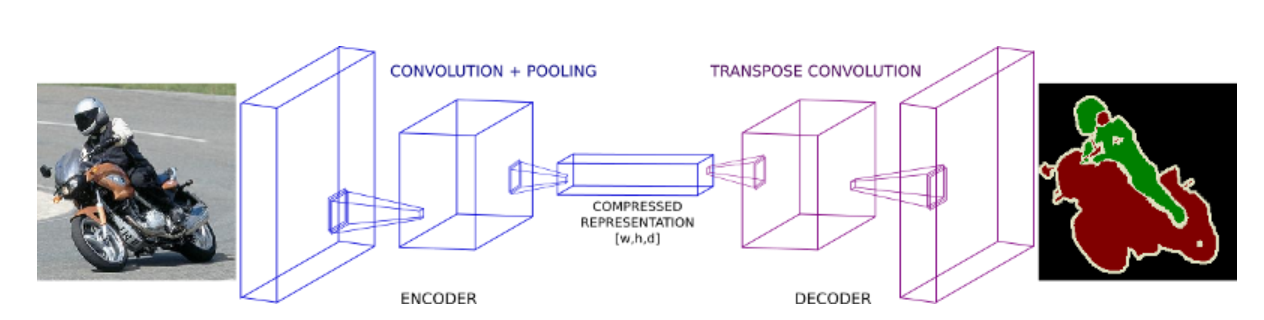图1.图像分割的下采样和上采样网络

转置卷积操作

现假设有一输入尺寸为2x2，转置卷积核大小为2x2，需要上采样到3x3，如图2所示，图改自图源。将输入左上角的值0与内核相乘，得到的2x2的张量放输出的左上角（红框区域），将输入右上角的值1与内核相乘，得到的2x2的张量放输出的右上角（蓝框区域），将输入左下角的值2与内核相乘，得到的2x2的张量放输出的左下角（黄框区域），将输入右下角的值3与内核相乘，得到的2x2的张量放输出的右下角（灰区域），重叠部分的值相加就得到了转置卷积的结果。
（CSDN意疏原创笔记：https://blog.csdn.net/sinat_35907936/article/details/107833112）图2.输入、内核与输出尺寸
上述转置卷积过程可以通过图3清晰表达出来，图源。观其过程，输入的每一个神经元都与输出的每4个神经元相连，并且共享一个卷积核，操作上的确是卷积反过来。从图1中解码部分示意图中也可以瞥见一斑。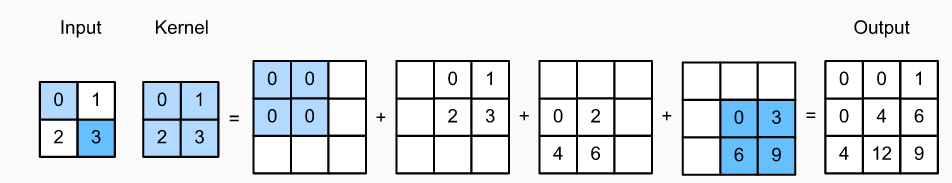图3.转置卷积过程

所以，转置卷积的输出尺寸公式，就应是卷积输出公式的反函数，如式（1）。输出尺寸w1、输入尺寸w，卷积核大小f，缩减大小p和步长s。padding变成了减，故有padding时应该将输入向中心缩小。s(w-1)，可以知道当有stride时，是将输入做膨胀，与dilation类似。两者都是卷积处操作的反过程。上述的运算过程可以通过卷积运算来完成，将内核旋转180度，然后与输入做卷积，保证输入与内核至少有一个元素相交，不相交部分输入补零。过程如图4所示，图改自图源。图4.转置卷积变卷积

Pytorch转置卷积层

Pytorch的转置卷积层有三个，分别是nn.ConvTranspose1d()、nn.ConvTranspose2d()和nn.ConvTranspose3d()。三者的操作过程与参数都是相同的，所以以下也只单独学习最最常用的nn.ConvTranspose2d()。

nn.ConvTranspose2d()

CLASS torch.nn.ConvTranspose2d(in_channels: int,
out_channels: int,
kernel_size: Union[int, Tuple[int, int]],
stride: Union[int, Tuple[int, int]] = 1,
padding: Union[int, Tuple[int, int]] = 0,
output_padding: Union[int, Tuple[int, int]] = 0,
groups: int = 1,
bias: bool = True,
dilation: int = 1,
padding_mode: str = 'zeros')

容易发现转置卷积层的参数与卷积层的参数很多是一致的，没有差异的参数将不赘述，详见此文。 以下只列出有差异的参数。用1x1的kernel时，可以由输入输出之间的关系，来间接反应这些参数的影响，以此来验证式（1）处的分析。
代码：
import torch
import torch.nn as nn

in_tensor = torch.tensor([[1, 2, 3, 4, 5],
[1, 2, 3, 4, 5],
[1, 2, 3, 4, 5],
[1, 2, 3, 4, 5],
[1, 2, 3, 4, 5]], dtype=torch.float)
in_tensor = torch.reshape(in_tensor, [1, 1, 5, 5])              # 转到四维
print(in_tensor)
deconv1 = nn.ConvTranspose2d(1, 1, (1, 1), bias=False, stride=1)
deconv1.weight.data = torch.tensor([[[]]], dtype=torch.float)
out_tensor = deconv1(in_tensor)
print(out_tensor)

# CSDN意疏原创笔记：https://blog.csdn.net/sinat_35907936/article/details/107833112

stride: 当stride=1、padding=0、kernel=1*1并且权值为1时，由图5方法可得输出应该和输入一模一样。代码验证后发现的确如此。维持其他参数不变，让stride=2时，可以发现输出是在输入的每两个元素间都插入了一个0的结果，此时两个相邻非零元素的间距是2。维持其他参数不变，让stride=3时，可以发现输出是在输入的每两个元素间都插入了两个0的结果，此时两个相邻非零元素的间距是3…。可见转置卷积层的stride，就等价于在input每两个元素之间插入(stride-1)个0，即将输入膨胀。
结果：
# input
tensor([[[[1., 2., 3., 4., 5.],
[1., 2., 3., 4., 5.],
[1., 2., 3., 4., 5.],
[1., 2., 3., 4., 5.],
[1., 2., 3., 4., 5.]]]])

# stride = 1  padding = 0
tensor([[[[1., 2., 3., 4., 5.],
[1., 2., 3., 4., 5.],
[1., 2., 3., 4., 5.],
[1., 2., 3., 4., 5.],
[1., 2., 3., 4., 5.]]]], grad_fn=<SlowConvTranspose2DBackward>)

# stride = 2  padding = 0
tensor([[[[1., 0., 2., 0., 3., 0., 4., 0., 5.],
[0., 0., 0., 0., 0., 0., 0., 0., 0.],
[1., 0., 2., 0., 3., 0., 4., 0., 5.],
[0., 0., 0., 0., 0., 0., 0., 0., 0.],
[1., 0., 2., 0., 3., 0., 4., 0., 5.],
[0., 0., 0., 0., 0., 0., 0., 0., 0.],
[1., 0., 2., 0., 3., 0., 4., 0., 5.],
[0., 0., 0., 0., 0., 0., 0., 0., 0.],
[1., 0., 2., 0., 3., 0., 4., 0., 5.]]]],
grad_fn=<SlowConvTranspose2DBackward>)
# stride = 3  padding = 0
tensor([[[[1., 0., 0., 2., 0., 0., 3., 0., 0., 4., 0., 0., 5.],
[0., 0., 0., 0., 0., 0., 0., 0., 0., 0., 0., 0., 0.],
[0., 0., 0., 0., 0., 0., 0., 0., 0., 0., 0., 0., 0.],
[1., 0., 0., 2., 0., 0., 3., 0., 0., 4., 0., 0., 5.],
[0., 0., 0., 0., 0., 0., 0., 0., 0., 0., 0., 0., 0.],
[0., 0., 0., 0., 0., 0., 0., 0., 0., 0., 0., 0., 0.],
[1., 0., 0., 2., 0., 0., 3., 0., 0., 4., 0., 0., 5.],
[0., 0., 0., 0., 0., 0., 0., 0., 0., 0., 0., 0., 0.],
[0., 0., 0., 0., 0., 0., 0., 0., 0., 0., 0., 0., 0.],
[1., 0., 0., 2., 0., 0., 3., 0., 0., 4., 0., 0., 5.],
[0., 0., 0., 0., 0., 0., 0., 0., 0., 0., 0., 0., 0.],
[0., 0., 0., 0., 0., 0., 0., 0., 0., 0., 0., 0., 0.],
[1., 0., 0., 2., 0., 0., 3., 0., 0., 4., 0., 0., 5.]]]],
grad_fn=<SlowConvTranspose2DBackward>)

# CSDN意疏原创笔记：https://blog.csdn.net/sinat_35907936/article/details/107833112

padding： 与上面同样的输入和卷积核，让stride = 1，修改padding，padding=1时，输出是输入上下少一行，左右少一列的结果。padding=2时，输出是输入上下少两行行，左右少两行的结果。可见转置卷积层的padding，就等价于在input上，边缘处上下各去掉padding行，左右各去掉padding列的结果。即将输入向中心缩小。
# input
tensor([[[[1., 2., 3., 4., 5.],
[1., 2., 3., 4., 5.],
[1., 2., 3., 4., 5.],
[1., 2., 3., 4., 5.],
[1., 2., 3., 4., 5.]]]])

# padding = 1  stride = 1
tensor([[[[2., 3., 4.],
[2., 3., 4.],
[2., 3., 4.]]]], grad_fn=<SlowConvTranspose2DBackward>)

# padding = 2  stride = 1
tensor([[[[3.]]]], grad_fn=<SlowConvTranspose2DBackward>)

# CSDN意疏原创笔记：https://blog.csdn.net/sinat_35907936/article/details/107833112

output_padding： 默认在输出的最右边拓展output_padding列，在输出的最下边拓展output_padding行。output_padding必须比stride或者dilation小，否则会报错：RuntimeError: output padding must be smaller than either stride or dilation.
deconv1 = nn.ConvTranspose2d(1, 1, (1, 1), bias=False, stride=2, padding=0, output_padding=1)

结果：
# input
tensor([[[[1., 2., 3., 4., 5.],
[1., 2., 3., 4., 5.],
[1., 2., 3., 4., 5.],
[1., 2., 3., 4., 5.],
[1., 2., 3., 4., 5.]]]])

# stride=2, padding=0, output_padding=1
tensor([[[[1., 0., 2., 0., 3., 0., 4., 0., 5., 0.],
[0., 0., 0., 0., 0., 0., 0., 0., 0., 0.],
[1., 0., 2., 0., 3., 0., 4., 0., 5., 0.],
[0., 0., 0., 0., 0., 0., 0., 0., 0., 0.],
[1., 0., 2., 0., 3., 0., 4., 0., 5., 0.],
[0., 0., 0., 0., 0., 0., 0., 0., 0., 0.],
[1., 0., 2., 0., 3., 0., 4., 0., 5., 0.],
[0., 0., 0., 0., 0., 0., 0., 0., 0., 0.],
[1., 0., 2., 0., 3., 0., 4., 0., 5., 0.],
[0., 0., 0., 0., 0., 0., 0., 0., 0., 0.]]]],
grad_fn=<SlowConvTranspose2DBackward>)

# CSDN意疏原创笔记：https://blog.csdn.net/sinat_35907936/article/details/107833112

加上dilation，output_padding后的转置卷积输出尺寸公式如下所示。其中，W指输出的宽，H指输出的高。

H out =(H in−1)×stride−2×padding+dilation×(kernel_size−1)+output_padding+1
W out =(W in−1)×stride−2×padding+dilation×(kernel_size−1)+output_padding+1

nn.ConvTranspose2d()实现

转置卷积同样需要转矩阵乘法来实现，如图5所示，其改自国外一篇文章：Up-sampling with Transposed Convolution。与卷积一样，转置卷积实现的关键也在于产生稀疏矩阵（sparse matrix），将稀疏矩阵与输入内积的结果resize就可以得到转置卷积的输出。图5.转置卷积转矩阵乘法

稀疏矩阵D（sparse matrix D） 也是由被flatten的转置卷积核叠放而成的，与卷积层那里有相似之处。剔除卷积核中的某些元素，让其和输入尺寸一致，然后再fatten成一维张量。由图4的方法，我们可以知道应该保留转置卷积核的哪些值，该剔除哪些值，如图6所示，其改自国外一篇文章：Up-sampling with Transposed Convolution。由于输出是4X4，故应该叠16层。图6.转置卷积稀疏矩阵

回忆卷积层处的稀疏矩阵C，当转置卷积与卷积的输入输出尺寸是互换的，并且相同卷积核时，转置卷积形成的稀疏矩阵D与卷积形成的稀疏矩阵C是转置的关系，不同卷积核时，如果只看元素位置，不看元素大小，也是转置的关系，这也是转置卷积名称的来源，如图7所示，其改自国外一篇文章：Up-sampling with Transposed Convolution。所以我们可以用产生稀疏矩阵C的方法，来间接产生稀疏矩阵D，以简化产生过程。图7.转置卷积稀疏矩阵与卷积稀疏矩阵的关系

nn.ConvTranspose2d()应用

在设计时，一般我们是知晓输入和输出尺寸的，而我们需要推算的应该是stride、padding和卷积核的大小。以上一篇笔记中卷积得到的438x438的特征图作为输入。输出440x440的图像，由于只放大了一点点，故stride=1，在不用空洞，不设dilation，output_padding的情况下，则440=437-2*padding+kernel_size，如果kernel_size=3，则padding=0，若kernel_size=4，则padding=1。
取stride=1，padding=0,kernel_size=4这正好是先前卷积过程的反过程。
代码：transform_inverse()函数定义见此文
import torch
import torch.nn as nn
from PIL import Image
import matplotlib.pyplot as plt
import torchvision.transforms as transforms
from tools.transform_inverse import transform_inverse

pil_img = Image.open('data/lenna.jpg').convert('L')
img = transforms.ToTensor()(pil_img)
c = img.size()
h = img.size()
w = img.size()
input_img = torch.reshape(img, [1, c, h, w])  # 转换成4维,[batch_size, c, h, w]
print(input_img.size())

# 卷积层
conv1 = nn.Conv2d(1, 2, (3, 3), bias=False)   # 实例化
conv1.weight.data = torch.tensor([[1, 2, 1],
[0, 0, 0],
[-1, -2, -1]])  # 水平边缘的sobel算子
conv1.weight.data = torch.tensor([[-1, 0, 1],
[-2, 0, 2],
[-1, 0, 1]])    # 竖直边缘的sobel算子
# print(conv1.weight.data)

out_img = conv1(input_img)                           # 两张特征图
print(out_img.size())
out_img = torch.squeeze(out_img, dim=0)
out_img_ = out_img
out_img = out_img+out_img                      # 水平边缘加竖直边缘等于全部边缘
out_pil_img = transform_inverse(torch.reshape(out_img, [1, out_img.size(), out_img.size()]), None)
plt.figure(0)
ax = plt.subplot(2, 2, 1)
ax.set_title('conv input picture')
ax.imshow(pil_img, cmap='gray')
ax = plt.subplot(2, 2, 2)
ax.set_title('conv output picture')
ax.imshow(out_pil_img, cmap='gray')

# CSDN意疏原创笔记：https://blog.csdn.net/sinat_35907936/article/details/107833112
# 转置卷积层
deconv1 = nn.ConvTranspose2d(2, 1, (3, 3), bias=False)
deconv1.weight.data = torch.tensor([[1, 2, 1],
[0, 0, 0],
[-1, -2, -1]])  # 水平边缘的sobel算子
deconv1.weight.data = torch.tensor([[-1, 0, 1],
[-2, 0, 2],
[-1, 0, 1]])    # 竖直边缘的sobel算子
de_out = deconv1(torch.reshape(out_img_, [1, out_img_.size(),
out_img_.size(), out_img_.size()]))
de_out = torch.squeeze(de_out, dim=0)
print(de_out.size())
de_out_pil_img = transform_inverse(de_out, None)
ax = plt.subplot(2, 2, 3)
ax.set_title('deconv input picture')
ax.imshow(out_pil_img, cmap='gray')
ax = plt.subplot(2, 2, 4)
ax.set_title('deconv output picture')
ax.imshow(de_out_pil_img, cmap='gray')
plt.show()

# CSDN意疏原创笔记：https://blog.csdn.net/sinat_35907936/article/details/107833112

结果：经过上采样，细节更丰富了，但并没有恢复原图，如图8所示。可见转置卷积只可以被看做是卷积的反过程，而不是卷积的逆。https://towardsdatascience.com/transposed-convolution-demystified-84ca81b4baba ↩︎


展开全文• 1.关于pytorch中的upsample、反卷积的解释： https://blog.csdn.net/g11d111/article/details/82855946 2.关于反卷积与上采样的区别 https://www.zhihu.com/question/63890195 3.关于双线性差值的实现 ...
• pytorch 不使用转置卷积实现上采样 上采样（upsampling）一般包括2种方式： Resize，如双线性插值直接缩放，类似于图像缩放，概念可见最邻近插值算法和双线性插值算法——图像缩放 Deconvolution，也叫Transposed ...
• 一，FCN网络 FCN大致上就是下图这个结构： 原图通过“编码器网络”把图片越缩越小，然后再通过“解码器网络”把图片再进行逐步放大。...在语义分割中，必须对反卷积反卷积核进行参数初始化(这点...
• 在阅读YOLO模型和DenseNet网络模型的时候，...文章目录上采样（Upsample）反卷积（Transposed Convolution）pytorch实现上采样 上采样（Upsample） 在介绍上采样前，还有一个概念叫做下采样。下采样简单来讲，就是在卷深度学习 python 神经网络
• Fast Neural Style Transfer 1、简介 在原始风格迁移中，是以一张图片作为参数来训练它。...此外，网络的上采样，采用的是先放大图片再卷积的形式，而非反卷积。 于是构建出网络： class ResBlock(nn.Modul神经网络 深度学习 网络
• Net-带有可选的反卷积和batchnorm Net-具有多个resnet后端 使用Maxpool索引进行分池 即将来临 实现了DataLoader 要求 pytorch> = 0.4.0 火炬视觉== 0.2.0 科学的 tqdm 张量板 一线安装 pip install -r
• 因为对卷积运算有基础，就不从0开始写了，下面概括地介绍相关概念后，重点放在卷积层在PyTorch实现上。 卷积运算：卷积核在输入信号（图像）上滑动，相应位置上进行乘加。网上有很多图例，清晰地介绍了卷积运算的...深度学习 神经网络
• 基于深度图像先验（DIP）的动机，我们在本文中提出了两个生成网络，分别用于对清洁图像和模糊核的深度先验进行建模，并提出了一种针对盲反卷积的无约束神经优化解决方案（SelfDeblur）。 实验结果表明，与基准...
• 下面列出一些学习过程中的资料，资料列表会不断跟新 1、PyTorch学习笔记(11)——论nn.Conv2d中...2、卷积原理：几种常用的卷积（标准卷积、深度卷积、组卷积、扩展卷积、反卷积） 3、PyTorch底层组卷积的实现方式 ...
• 或者是其他的信号，经过一系列操作，比如卷积，或者linear变换，变换得到一个向量，这个向量就叫做对这个图像的编码，这个过程就叫做encoder，对于一个特定的编码，经过一系列反卷积或者是线性变换，得到一副图像，...
• 因为最近在学习FCN，所以...2：Deconvolution，也叫Transposed Convolution，也叫做反卷积。 一：Resize CLASS torch.nn.Upsample(size=None, scale_factor=None, mode='nearest', align_corners=None) CLASS torch.nn上采样
• 在此程序包中，我基于torch.nn.Module在PyTorch实现了NMF，PLC​​A及其反卷积变化，因此可以在CPU / GPU设备之间自由移动模型并利用cuda的并行计算。 模组 NMF 基本的NMF和NMFD模块使用乘法更新规则将beta差异...
• 使用对抗式生成网络基于MNIST的手写数字数据集实现自动生成手写数字，基于pytorch实现。 数据集来源：Kaggle数据集 部分训练结构参考来源：GAN生成MNIST数据集（pytorch版） 测试了一些其它博客中的代码，但是发现很...深度学习 神经网络 机器学习
• 因为网络经过pooling、带stride的convolution等操作，相邻的偶数、奇数规模的输入可能得到了相同规模中间层，最后反卷积的结果不一定和输入规模相同，可以加入upsample的层，使用插值的方式和输入同规模的结果。...
• 1、export of nn.interpolate #pytroch Unsample 相当于 resize,nearst，不是反卷积实现的操作,下面是讨论 https://github.com/pytorch/pytorch/issues/34718 2、 该博客下的 pytorch1.0,1.0.1-- onnx --tensorRT5.0...
• ## 卷积的矩阵理解

千次阅读 2019-02-25 17:46:14
但是在代码实现的时候通常需要更为数学化的卷积表达形式，而且理解卷积的数学形式过来可以帮助更好地理解卷积操作的本质。 卷积的数学形式通常通过矩阵乘法来表示。本文从卷积最一般的数学形式开始讲起，并从一般...矩阵 神经网络
• 主要介绍卷积与反卷积神经网络的基本知识，大小的计算，以及如何实现人工智能 深度学习
• 看了下fcn的代码和理论。这里就以FCN16s为例讲解，16s清晰了 32s 8s 只是在...FCN16s说白了就是 将 1/32图的预测结果做反卷积，变成1 /16图。然后再将1 /16图池化结果做预测，并与前的1/32图 结果做加法。最终得到...
• znxlwm 使用InfoGAN的结构，卷积反卷积 eriklindernoren 把mnist转成1维，label用了embedding wiseodd 直接从tensorflow代码转换过来的，数据集居然还用tf的数据集。。 Yangyangii 转1维向量，全连接 FangYang970206...
• 1、其中再语义分割比较常用的上采样： 其实现方法为： def upconv2x2(in_channels, out_channels, ...这里时反卷积操作。 return nn.ConvTranspose2d( in_channels, out_channels, kernel_size=2, stride=2) els
• 本节第一部分学习卷积神经网络中最重要的卷积层，了解卷积操作的过程与步骤，同时学会区分一维/二维/三维卷积，最后学习转置卷积（Transpose Convolution）的由来以及实现方法； 本节第二部分学习池化层，全连接层...
• 本节第一部分学习卷积神经网络中最重要的卷积层，了解卷积操作的过程与步骤，同时学会区分一维/二维/三维卷积，最后学习转置卷积（Transpose Convolution）的由来以及实现方法； 本节第二部分学习池化层，全连接层...
• 本节第一部分学习卷积神经网络中最重要的卷积层，了解卷积操作的过程与步骤，同时学会区分一维/二维/三维卷积，最后学习转置卷积（Transpose Convolution）的由来以及实现方法； 本节第二部分学习池化层，全连接层和...
• PyTorch实现，用于单图像非盲去模糊。 数据 从下载综合数据集 预训练模型 从下载ADM的Z模块和UDN模型 跑步 请看一下demo.ipynb ，其中我们提供了Set14 / Levin数据集中的8个非盲反卷积示例，扰动的噪声水平可以从1...Python